# DC Circuit Analysis Loop Equations

All of the rules governing DC circuits that have been discussed so far can now be applied to analyze complex DC circuits. To apply these rules effectively, loop equations, node equations, and equivalent resistances must be used.

#### Loop Equations

As we have already learned, Kirchhoff’s Laws provide a practical means to solve for unknowns in a circuit. Kirchhoff’s current law states that at any junction point in a circuit, the current arriving is equal to the current leaving. In a series circuit the current is the same at all points in that circuit. In parallel circuits, the total current is equal to the sum of the currents in each branch. Kirchhoff’s voltage law states that the sum of all potential differences in a closed loop equals zero.

Using Kirchhoff’s laws, it is possible to take a circuit with two loops and several power sources (Figure 37) and determine loop equations, solve loop currents, and solve individual element currents.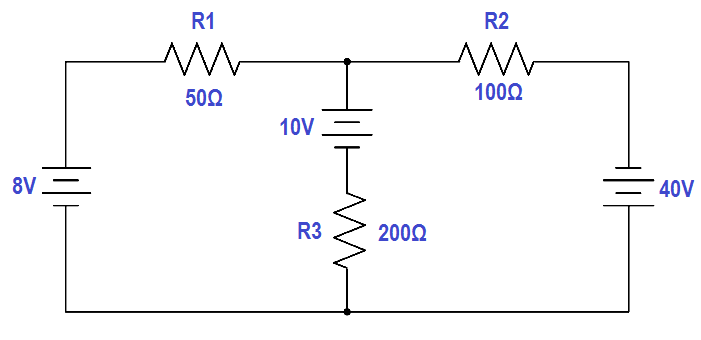Figure 37 Example Circuit for Loop Equations

The first step is to draw an assumed direction of current flow (Figure 38). It does not matter whether the direction is correct. If it is wrong, the resulting value for current will be negative.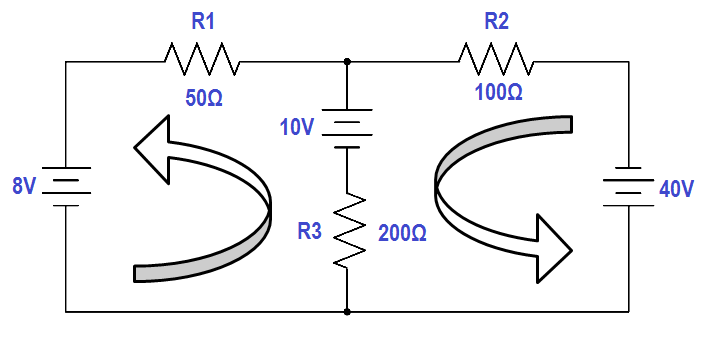Figure 38 Assumed Direction of Current Flow

Second, mark the polarity of voltage across each component (Figure 39). It is necessary to choose a direction for current through the center leg, but it is not necessary to put in a new variable. It is simply I2 – I1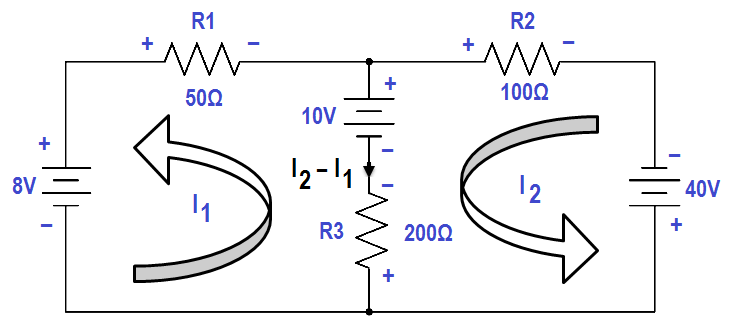Figure 39 Marking Polarity

Third, apply Kirchhoff’s voltage law to loops one and two by picking a point in each loop and writing a loop equation of the voltage drops around the loop; then set the equation equal to zero.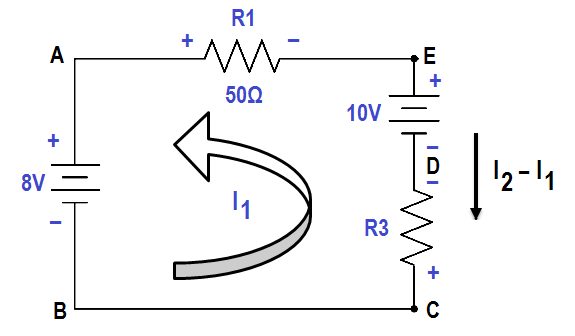Figure 40 Applying Voltage Law to Loop 1

Figure 40 shows Loop one. From Point A to Point B, there is an increase in voltage of 8 volts. From Point C to Point D, there is an increase in voltage of 200 (I2 -I1). From Point D to Point E, there is a decrease in voltage of 10 volts. From Point E to Point A, there is a voltage decrease of 50I1 volts. The result in equation form is illustrated in below equation 1.

8 + 200 (I2 – I1) – 50I1 – 10 = 0

Using the same procedure for Loop 2 of Figure 39, the resulting equation is shown in below equation 2

10 – 200 (I2 – I1) + 40 – 100I1 = 0

Fourth, solve equations (1) and (2) simultaneously. First, rearrange and combine like terms in the equation for Loop 1.

-50 I1 + 200 I2 – 200 I1 = 10 – 8

-250 I1 + 200 I2 =2

Divide both sides by two.

-125 I1 + 100 I2 = 1

Rearrange and combine like terms in the Loop 2 equation.

-200 I2 + 200 I1 – 100 I2 = -10 -40

200 I1 – 300 I= -50

Multiplying the Loop 1 equation by 3,

3 (-125 I1 + 100 I2 = 1 )

and add it to the Loop 2 equation.

-375 I1 + 300 I2 = 3

200 I1 – 300 I= -50

– – – – – – – – – – – – – – – – –

– 175 I1 + 0 = – 47

– – – – – – – – – – – – – – – – –

Solving for I1 :

– 175 I1 = – 47

I1 = – 47 / -175 = 0.2686 amp = 286.3 mA

Solving for I2 using the Loop 1 equation:

– 125 (0.2686) + 100 I2 = 1

100 I2 = 1 + 33.58

I2 = 0.3458 amp = 345.8 mA

The current flow through R1 (50Ω) is I1 . The current flow through R2 (100Ω) is I2 , and through R3 (200Ω) is I2 -I1

I3 = I2 – I1

I3 = 345.8 mA – 286.3 mA = 77.2 mA

Fifth, apply Ohm’s Law to obtain the voltage drops across Resistors R1, R2 , and R3 :

V1 = I1R1 = (0.2686 amps)(50Ω) = 13.43 Volts
V2 = I2R2 = (0.3458 amps)(l00Ω) = 34.58 Volts
V3 = (I2 – I1) R3 = (0.0772 amps)(200Ω) = 15.44 Volts

Sixth, check the calculations by applying Kirchhoff’s Laws:

Check 1: Apply Kirchhoff’s voltage law to the larger outer loop (Figure 41).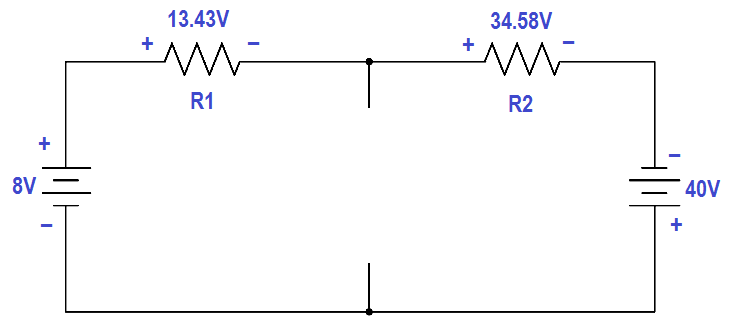Figure 41 Applying Voltage Laws to Outer Loop

The sum of the voltage drops around the loop is essentially zero. (Not exactly zero due to rounding off.)

8 – 13.43 – 34.58 + 40 = 0

0.01 ≅ 0

Therefore, the solution checks.

Check 2: Use Kirchhoff’s current law at one of the junctions (Figure 42).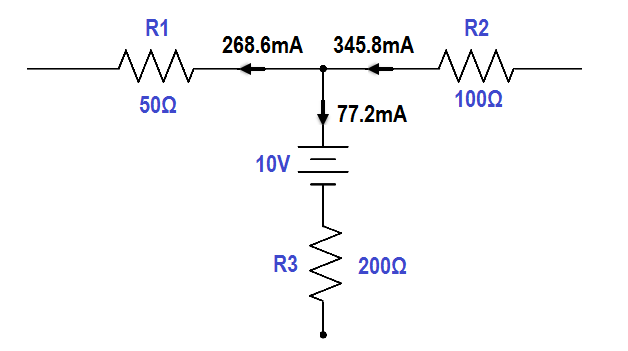Figure 42 Applying Current Law to Junction

The sum of the currents out of the junction is:

0.2686 + 0.0772 = 0.3458 A = 345.8 mA

The current into the junction is 345.8 mA

The current into the junction is equal to the current out of the junction. Therefore, the solution checks.